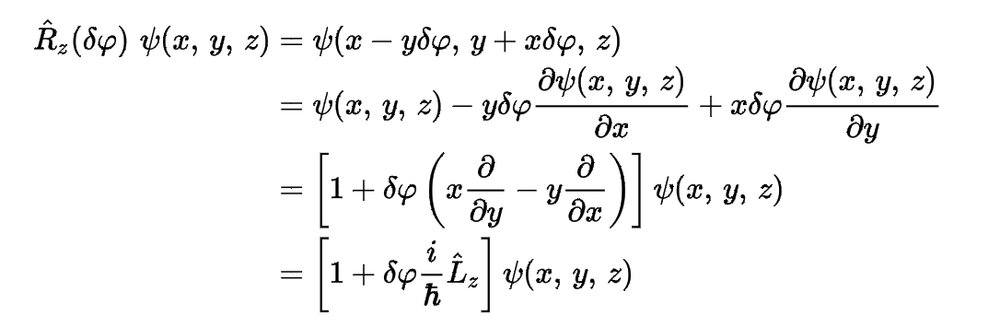# Rotation of functions

• I
Oliver321
TL;DR Summary
How is it possible to rotate a function (for example the 3D wave function) by an infinitesimal angle by using derivatives?
I was solving a problem for my quantum mechanics homework, and was therefore browsing in the internet for further information. Then I stumbled upon this here:R is the rotation operator, δφ an infinitesimal angle and Ψ is the wave function.
I know that it is able to rotate a curve, vector... with a rotation matrix. But how is it possible to rotate a function only with derivatives? I tried to rephrase a function f(x) as a curve, applying the 2D rotation matrix and small angle approximation and convert it back to an explicit function f(x). But I did not get the same answer.
My question is now: how does this work and what’s the connection to the rotation matrix?

I am really thankful for every help!

•jk22

•